’╗┐ Varipend┬«contents: Contacts     Engl   Find: in varipend.narod.ru Narod.ru Yandex About an opportunity of "reactionless" moving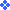2. Article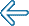1  2  3  4  5  6  7  8  9  10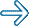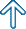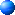2.5. Group work. Association of several systems of mobile elements. Process of moving of the considered system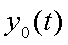it is possible to repeat. For this purpose it is required to move mass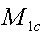, which gathers on distance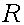from the center of mass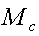, in the center of mass. Not breaking position of the center of mass of all system, in regular intervals to disperse system of mobile elementson a circle of radius. To give to system of mobile elements angular speed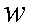. After that, it is possible to repeat a running cycle (a Fig. 25,Fig. 26). To exclude from calculations coordinate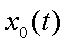and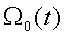(whereŚ a corner of turn of all mechanical system), it is convenient to bring in the closed mechanical system one more system of the mobile elements making movement, mirror symmetric to the first system of mobile elements concerning an axis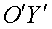.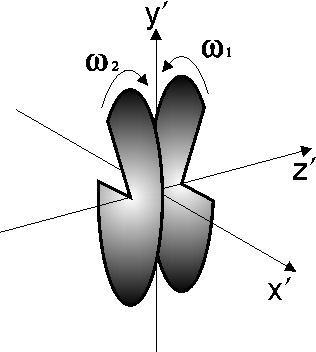Fig. 13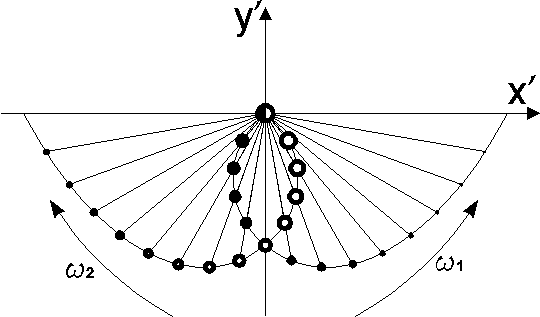Fig. 14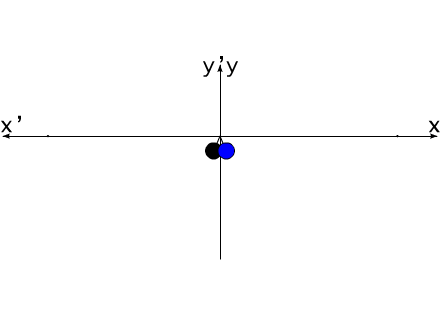It is meant mirror symmetry of movement: starting corner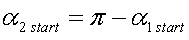angular speed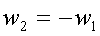change of mass of mobile elements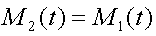elements of mobile system stop in a point with coordinates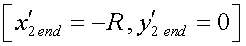Coordinates of the center of mass of the second mobile systemIn a projection to axes of  coordinates system XOY a linear momentum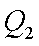:Total momentum of systems of mobile elements in a projection to an axis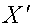it is equal to zero.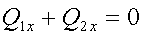Total momentum of two systems of mobile elements in a projection to an axis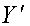it is equal to the double momentum of one of systems of mobile elements.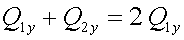The sum of the moments of momentums of two systems of mobile elements is equal to zero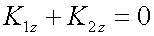As it was already mentioned above (Fig. 3), to coordinates system XOY it is possible to compare moving of the center of mass of system of mobile elements to moving the center of mass of a pendulum of variable length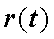and variable mass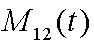.  For a case of two systems of mobile elements, moving of their general center of mass can be presented as back and forth motion of a body of variable mass on one coordinate. (Fig. 15). That is, the mechanical system consisting of two systems of mobile elements, possesses one degree of freedom.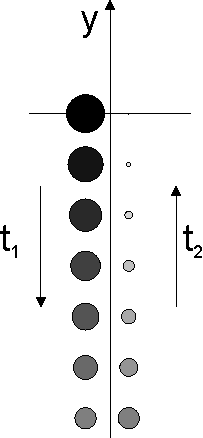Fig. 15   Animated image Fig. 15 :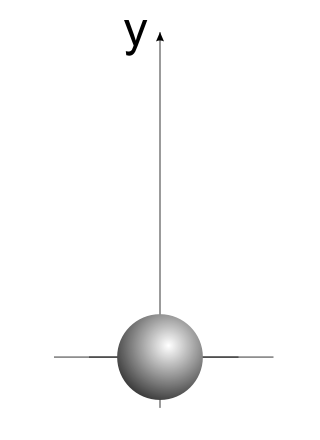During the first half-cyclethere is a reduction of mass of two systems of mobile elements. Thus coordinate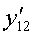(the center of mass of systems of mobile elements) decreases. During the second half-cycle, coordinateincreases, but mass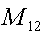continues to decrease up to zero value.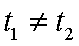(see Fig. 8)1  2  3  4  5  6  7  8  9  10// //
ąĪą░ą╣čé čüąŠąĘą┤ą░ąĮ ą▓ čüąĖčüčéąĄą╝ąĄ uCoz